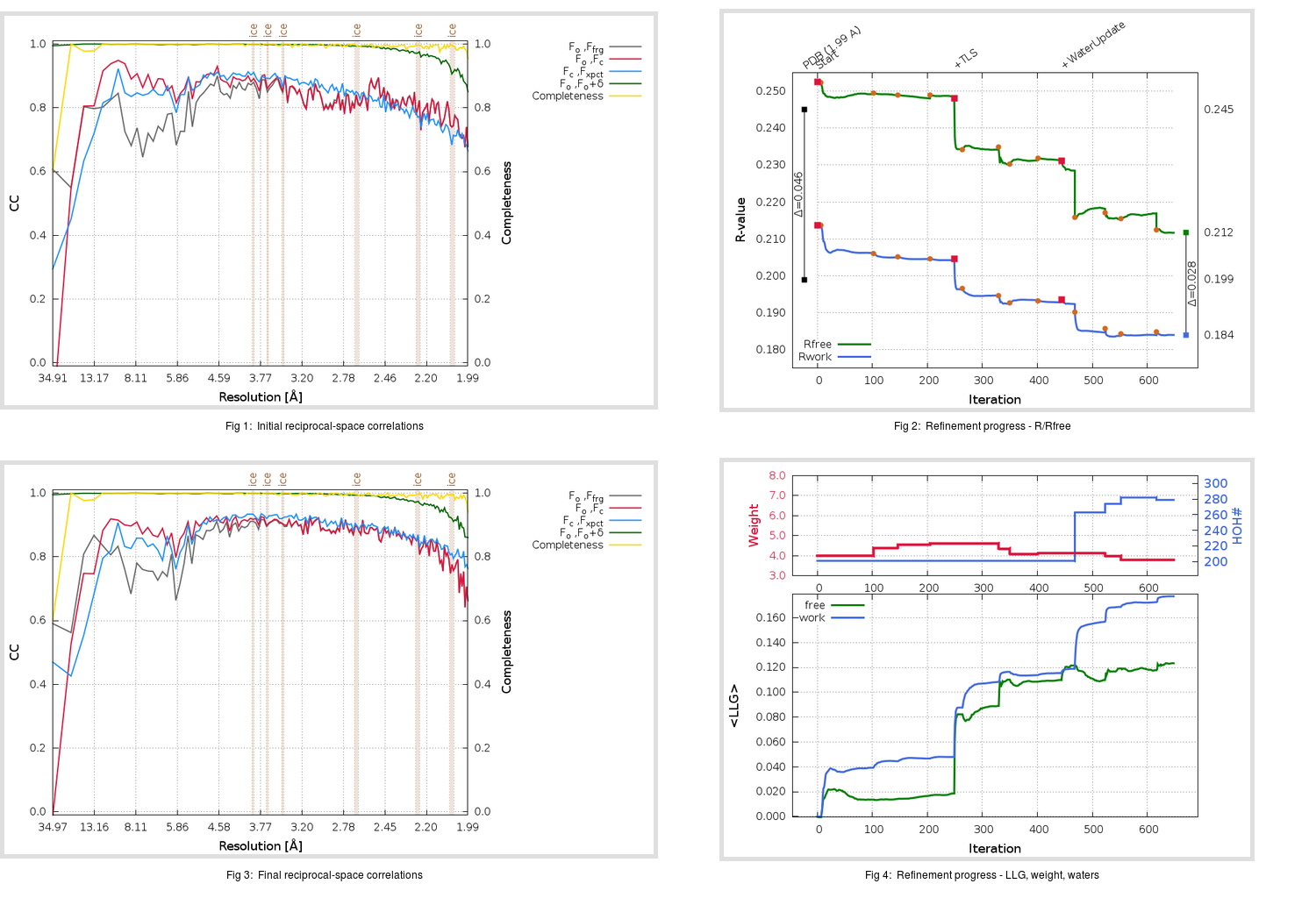Content:

```    Diffraction limits & principal axes of ellipsoid fitted to diffraction cut-off surface:
1.953         0.9591   0.0000   0.2832       a* + 0.016 c*
1.940         0.0000   1.0000   0.0000       b*
1.904        -0.2832   0.0000   0.9591      -0.495 a* + 0.869 c*
```

## Deposited

` `
 Date deposited Date data collection Resolution R, Rfree 20110901 20100806 1.99 0.1970 0.2450

Molprobity (CCP4 7.0 version) summary:

```Ramachandran outliers =   0.00 %
favored =  96.38 %
Rotamer outliers      =   0.76 %
C-beta deviations     =     0
Clashscore            =   5.84
RMS(bonds)            =   0.0147
RMS(angles)           =   1.43
MolProbity score      =   1.56
Resolution            =   1.99
R-work                =   0.1970
R-free                =   0.2450
```

```Number of waters      =   201

<B> (all atoms) =   29.95 ( sd =    9.00 ) for       2624 non-hydrogen atoms
<B>   (protein) =   30.22 ( sd =    8.98 ) for       2374 non-hydrogen atoms
<B>     (water) =   28.49 ( sd =    9.03 ) for        201 non-hydrogen atoms
<B>    (others) =   22.85 ( sd =    5.75 ) for         49 non-hydrogen atoms

B min/max       (all non-hydrogen atoms) =   11.52 /   65.97
B min/max   (protein non-hydrogen atoms) =   12.69 /   65.97
B min/max     (water non-hydrogen atoms) =   11.52 /   54.75
B min/max     (other non-hydrogen atoms) =   12.16 /   35.10
```

## BUSTER (re-)refinement

` `

Molprobity (CCP4 7.0 version) summary:

```Ramachandran outliers =   0.00 %
favored =  97.70 %
Rotamer outliers      =   0.38 %
C-beta deviations     =     0
Clashscore            =   3.54
RMS(bonds)            =   0.0121
RMS(angles)           =   1.55
MolProbity score      =   1.21
Resolution            =   1.99
R-work                =   0.1841
R-free                =   0.2117
```

```Number of waters      =   279

<B> (all atoms) =   33.66 ( sd =   10.96 ) for       2702 non-hydrogen atoms
<B>   (protein) =   32.31 ( sd =   10.15 ) for       2374 non-hydrogen atoms
<B>     (water) =   45.31 ( sd =   11.52 ) for        279 non-hydrogen atoms
<B>    (others) =   32.79 ( sd =    4.53 ) for         49 non-hydrogen atoms

B min/max       (all non-hydrogen atoms) =   15.48 /   77.80
B min/max   (protein non-hydrogen atoms) =   15.48 /   77.80
B min/max     (water non-hydrogen atoms) =   15.85 /   75.82
B min/max     (other non-hydrogen atoms) =   24.06 /   40.36
```

Refinement progression:Results:

` `
 File Remark 3TNS_aB_refine.01_03_refine.pdb.gz exact refinement commands are in header 3TNS_aB_refine.01_03_refine.mtz.gz including original deposited data and several re-refinement map coefficients 3TNS_aB_refine.01_03_BUSTER_model.cif.gz including any non-standard compound restraints 3TNS_aB_refine.01_03_BUSTER_refln.cif.gz